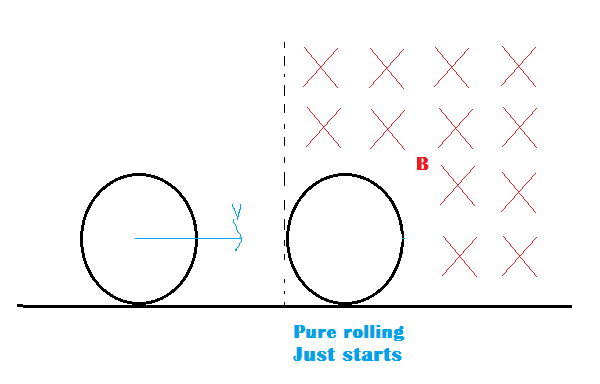# Rolls When Completely In!A ring of mass m and radius r and made of an insulating material carries uniform charge. Initially it rests vertically on a frictionless horizontal tabletop on the left side of the vertical plane AA'. In the region to the right side of AA' is a uniform horizontal magnetic field of induction B pointing everywhere parallel to the axis of the ring. The ring is pushed forward with velocity v but no rotation. If $q$ is the charge on the ring so that it just starts rolling on the tabletop on entering completely into the magnetic field, what is [200q] in SI units?

details and Assumptions

$\bullet$ In the given figure cross sign indicates that the field is towards us.

$\bullet$ neglect induced electric fields.

$\bullet$ the mass per unit length $\lambda$

$\bullet$ $\lambda=7$

$\bullet$ $v=3m/s$

$\bullet$ $B=4T$

×